Know your reciprocals by playing this quiz.

# Algebra - Reciprocals 1 - Easy

This Math quiz is called 'Algebra - Reciprocals 1 - Easy' and it has been written by teachers to help you if you are studying the subject at middle school. Playing educational quizzes is a fabulous way to learn if you are in the 6th, 7th or 8th grade - aged 11 to 14.

It costs only \$12.50 per month to play this quiz and over 3,500 others that help you with your school work. You can subscribe on the page at Join Us

What exactly is a reciprocal? A reciprocal is the inverse of a number or more accurately, the reverse of a fraction. As an example, take the fraction 3/4. The reciprocal of 3/4 is 4/3 as 4/3 is the inverse or reverse of 3/4. Now what would you do if you were asked to multiply a number by its reciprocal?

Let’s look at the above fraction of 3/4. We know that the reciprocal is the reverse so we would create a problem that reads:

3/4 x 4/3

Remember when multiplying fractions you multiply the numerators together and then you multiply the denominators together so that now you get:

3/4 x 4/3 = 12/12

12/12 is the same as “1”

A number when multiplied by its reciprocal will always equal “1”. In fact, any mathematical problem when multiplied by its reciprocal will always equal “1”.

Now what would you do if you were asked to find the reciprocal of a whole number and then multiply it by its reciprocal? Let’s say the number 10? How do you reverse 10? Well, 10 is actually the whole number of the fraction 10/1. Ah, now what would be its reciprocal? That’s right, 1/10. Now we can work the problem as follows:

10/1 x 1/10 = 10/10 = 1

Reciprocals seem to be very easy but let’s make sure you understand the concept. Below are ten problems or questions regarding reciprocals to see just how much you do know and where you might need to take a quick look back for more clarification.

1.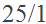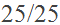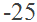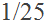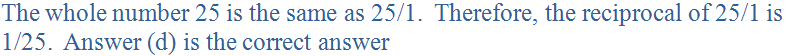2.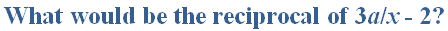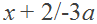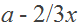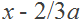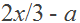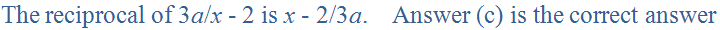3.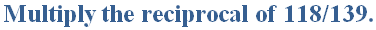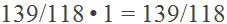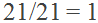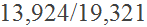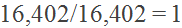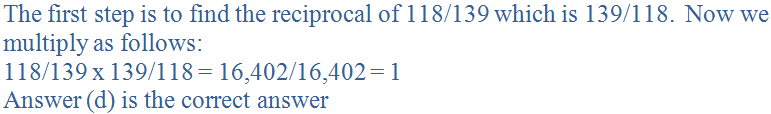4.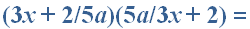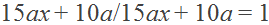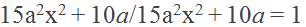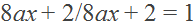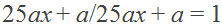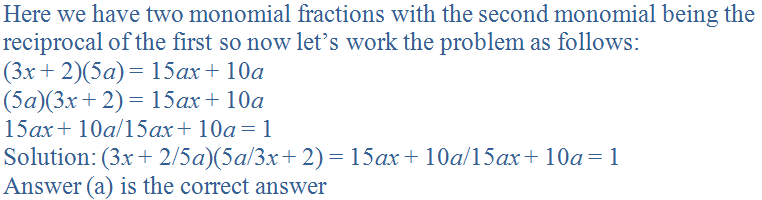5.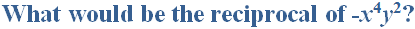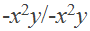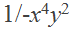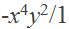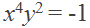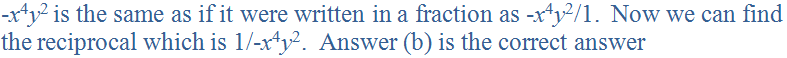6.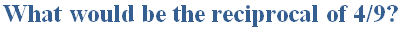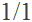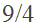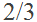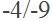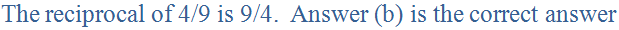7.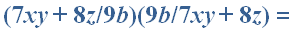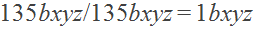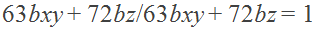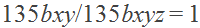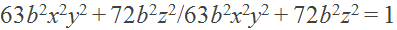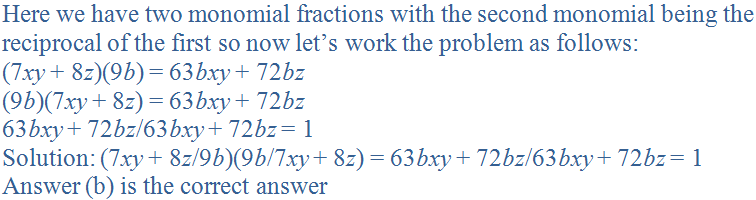8.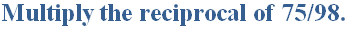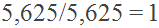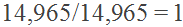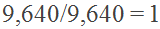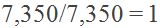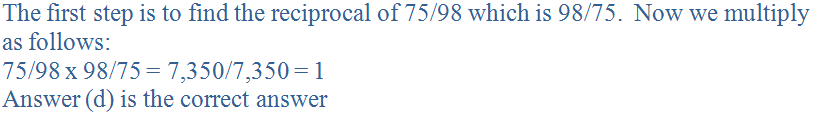9.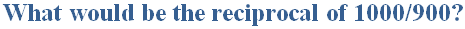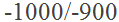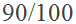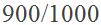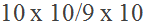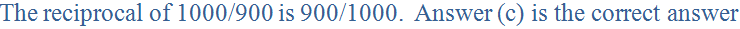10.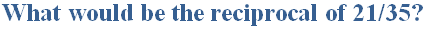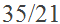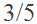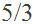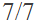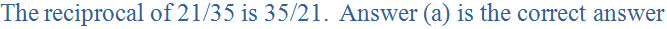Author:  Christine G. Broome